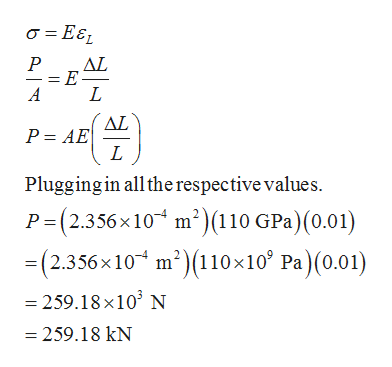# A hollow copper pipe undergoes a 1% change in length under a tensile load. If the Young’s modulus of copper is 110 GPa, the pipe’s outer diameter is 2 cm and its wall thickness is 5 mm, what is the magnitude of the tensile load? Consider the whole change happening in the elastic region.

Question
59 views

A hollow copper pipe undergoes a 1% change in length under a tensile load. If the Young’s modulus of copper is 110 GPa, the pipe’s outer diameter is 2 cm and its wall thickness is 5 mm, what is the magnitude of the tensile load? Consider the whole change happening in the elastic region.

check_circle

Step 1

We have been given the following data:

The percentage change in length of the hollow copper pipe, ΔL/L = 1% = 0.01.

The Young’s modulus of copper, E = 110 Gpa.

The outer diameter of the pipe, Do = 2 cm.

The wall thickness, t = 5 mm.

Step 2

Calculate the inner diameter of the pipe and the cross-sectional area of the pipe.

Step 3

Calculate the tensile load by applying the longitudinal stres...help_outlineImage TranscriptioncloseAL = E L A AL P AE L Plugging in allthe respective values. P (2.356x10 m2)(110 GPa)(0.01) =(2.356x 10 m2110x10 Pa) (0.01) -259.18 x103 N =259.18 kN fullscreen

### Want to see the full answer?

See Solution

#### Want to see this answer and more?

Solutions are written by subject experts who are available 24/7. Questions are typically answered within 1 hour.*

See Solution
*Response times may vary by subject and question.
Tagged in

### Mechanical Engineering